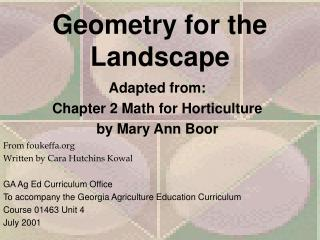DownloadDownload PresentationGeometry for the Landscape

# Geometry for the Landscape

Download Presentation## Geometry for the Landscape

- - - - - - - - - - - - - - - - - - - - - - - - - - - E N D - - - - - - - - - - - - - - - - - - - - - - - - - - -
##### Presentation Transcript

1. Geometry for the Landscape Adapted from: Chapter 2 Math for Horticulture by Mary Ann Boor From foukeffa.org Written by Cara Hutchins Kowal GA Ag Ed Curriculum Office To accompany the Georgia Agriculture Education Curriculum Course 01463 Unit 4 July 2001

2. Objectives • By the end of this lesson you will be able to calculate: • the perimeter of a given section of land • the area of given section of land • the volume of container

3. Terms • diameter (d)- the line that divides a circle into two equal parts • radius ( r)- one half the diameter • pi () - 3.14 or 22/7 • height- line segment that shows how tall a triangle is. It is always drawn from a corner of the triangle to the opposite side forming a 90 degree angle.

4. Terms continued • perimeter(P)- the distance around • the sum of all sides • How much fence do you need to go around a yard? • Circumference (C)- perimeter of a circle • C= diameter X  • How much edging do you need to go around a flower bed?

5. Terms Continued • volume (V)- how much fits in a given space • Volume of a box= area of bottom X height • Volume of a sphere+ 4/3 X pi X radius X radius X radius • How much soil do you need to fill a flower pot?

6. More Terms • area (A)- the space inside the perimeter • rectangle= side X side • triangle= (base X height)/2 • circle= radius X radius X  • How many square feet of sod do you need to order to cover the yard?

7. Example of perimeter 2 4 2 2 3 2 3 2+2+2+2=8 3+3+4=10

8. Examples of area rectangle 2 5 2 X 5 = 10

9. Examples of area circle 2 2 X 2 X 3.14= 12.56

10. Examples of Area of Triangle A=(8X6)/2 A=48/2 A=24 8.9 8.2 8 6

11. Volume Area of bottom=7'X7' Height=9' Volume=7'X7'X9' Volume=441 cubic feet 7' 7' 9'

12. Notes • If a shape is not standard, break it down into smaller standard shapes to make calculations • Calculations must be done in the same unit of measurement (change feet to inches or vice versa as needed) • Simplify all answers, do not write feet as decimals, convert to inches.

13. Units • Perimeter- feet, inches, cm, m, mm, yards……. • Area- sq. feet, sq. inches, sq. cm, sq. yards…... • Volume- cubic feet, cubic inches, cubic cm, cubic yards…….

14. Homework problems 1.How many cubic feet of mulch do you need to buy if you are mulching a 3 foot ring three inches deep around 4 trees that are 1 foot each in diameter? 2. How much soil is needed to fill 24 planters that are 1 ft wide, 6 feet long, and 1ft 6 inches deep?

15. 38’ 3. Find the perimeter of the yard to the right. 4. How many sq. feet of sod would be needed to cover the yard at the right? 72’

16. 5. If three inches of snow had to be removed from a walk pictured below, how many cubic ft. of snow would be removed? 46’ 12’ 50’ 8’